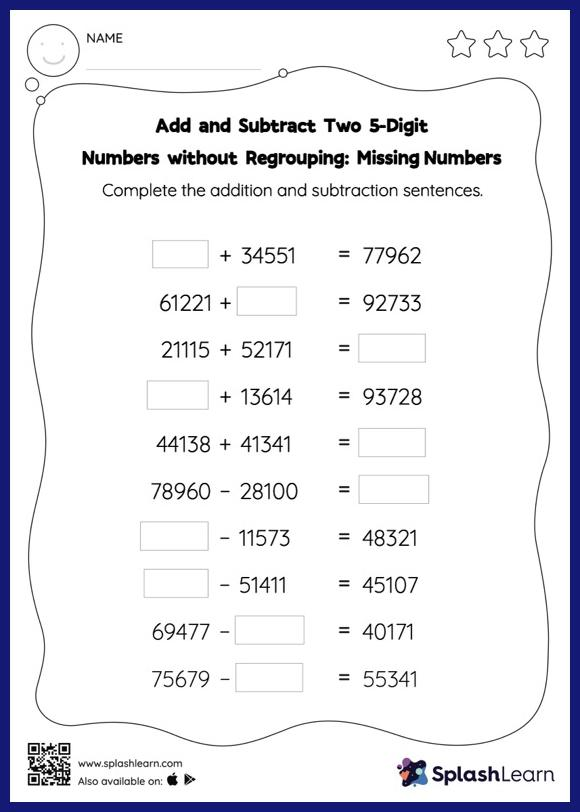# Add and Subtract Two 5-Digit Numbers without Regrouping: Missing Numbers Worksheet

Home > Add and Subtract Two 5-Digit Numbers without Regrouping: Missing NumbersIn this worksheet, students must find the missing number using the relationship between addition and subtraction. To solve add and subtract two 5-digit numbers without regrouping worksheet, they do not need to regroup the numbers. In each problem, the numbers are laid out in the horizontal format. Students should try to use different strategies involving composing and decomposing numbers to solve these problems. This will help them develop flexibility and fluency.